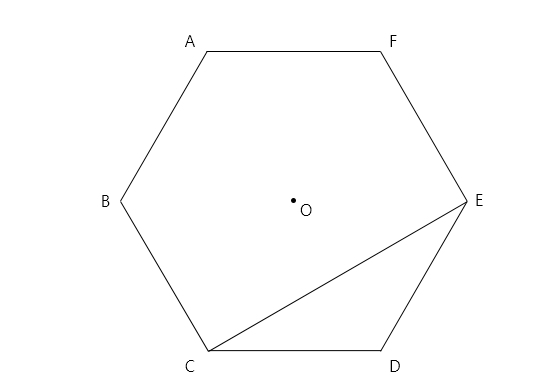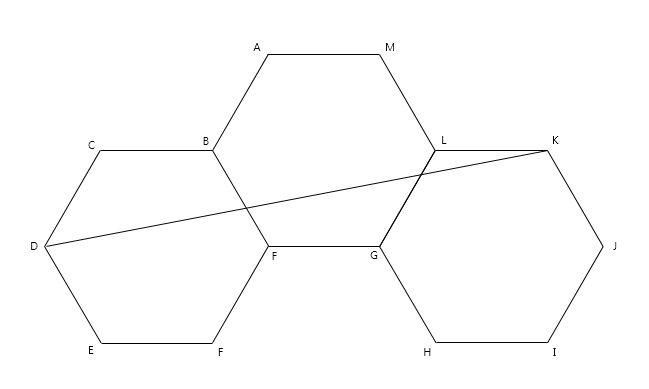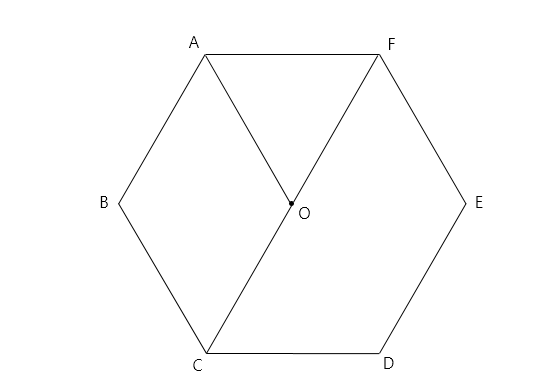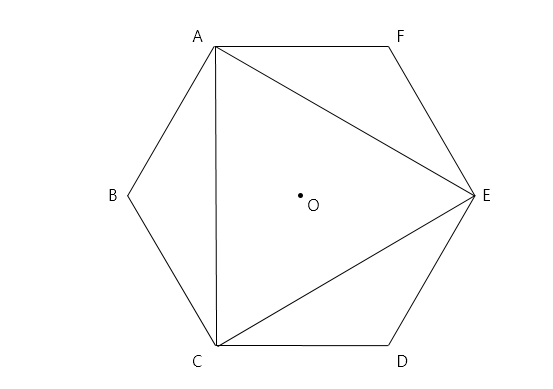Geometry

Regular Polygons - Properties of Regular HexagonsThe above diagram is a regular hexagon. If the length of $\overline {AB}$ is $19,$ what is the area of $\triangle CDE ?$The three hexagons in the above diagram are regular hexagons. If the side length of each hexagon is $23,$ what is the length of $\overline {DK} ?$

A regular hexagon has side length $8$ cm. What is the perimeter (in cm) of the hexagon?Point O is the center of the above regular hexagon. What is the area of quadrilateral $CDEF$ when the area of triangle $AOF$ is $3?$The above diagram is a regular hexagon. What is the area of $\triangle ACE$ when the side length of the regular hexagon is $21?$

×